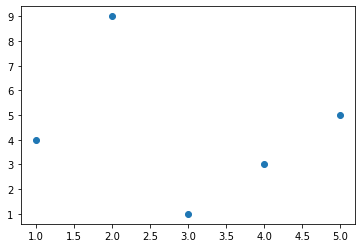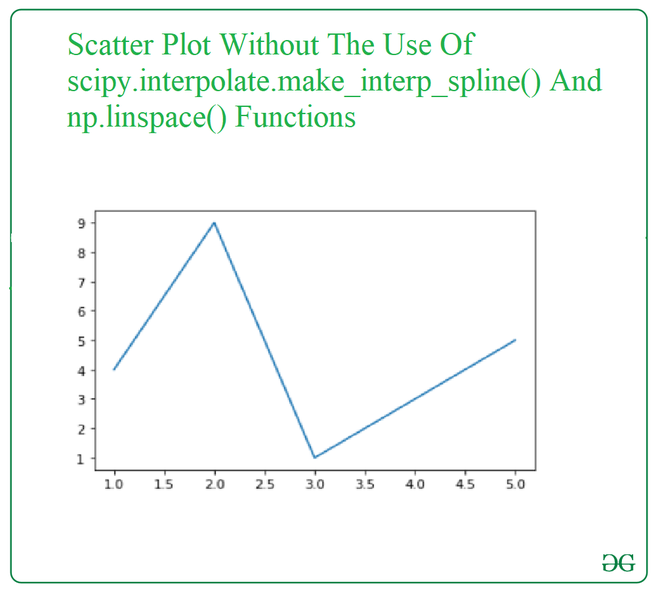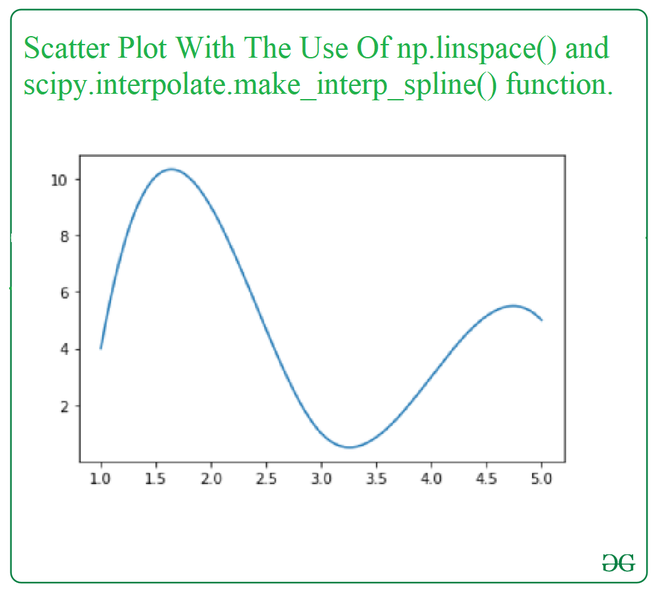# Create Scatter Plot with smooth Line using Python

A curve can be smoothened to reach a well approximated idea of the visualization. In this article, we will be plotting a scatter plot with the smooth line with the help of the SciPy library. To plot a smooth line scatter plot we use the following function:

• scipy.interpolate.make_interp_spline() from the SciPy library computes the coefficients of interpolating B-spline. By importing, this function from the Scipy library and added the parameter, It is quite easier to get the smooth line to scatter plot.

Syntax:

scipy.interpolate.make_interp_spline(x, y, k=3, t=None, bc_type=None, axis=0, check_finite=True)

Parameters:

• x:-Abscissas
• y:-Ordinates
• k:-B-spline degree
• t:-Knots
• bc_type:-Boundary conditions
• axis:-Interpolation axis
• check_finite:-Whether to check that the input arrays contain only finite numbers

Return: a BSpline object of the degree k and with knots t.

• np.linspace() function is imported from NumPy library used to get evenly spaced numbers over a specified interval used to draw a smooth line scatter plot.

Syntax:

numpy.linspace(start, stop, num=50, endpoint=True, retstep=False, dtype=None, axis=0)

Parameters:

• start:-The starting value of the sequence.
• stop:-The end value of the sequence.
• num:-Number of samples to generate.
• endpoint:-If True, stop is the last sample.
• retstep:-If True, return (samples, step), where the step is the spacing between samples.
• dtype:-The type of the output array.
• axis:- The axis in the result to store the samples.

Return: A array of num equally spaced samples in the closed interval

Approach

• Import module
• Create a scatter plot
• Create a smoothened curve from the points of the scatter plot
• Display plot

Example:

## Python3

 `import` `numpy as np ` `import` `matplotlib.pyplot as plt ` ` `  `x ``=` `np.array([``1``, ``2``, ``3``, ``4``, ``5``]) ` ` `  `y ``=` `np.array([``4``, ``9``, ``1``, ``3``, ``5``]) ` ` `  `plt.scatter(x, y) ` ` `  `plt.show() `

Output:Now let’s visualize the scatter plot by joining points of the plot so that an uneven curve can appear i.e. without smoothening so that difference can be apparent.

Example:

## Python3

 `import` `numpy as np ` `import` `matplotlib.pyplot as plt ` ` `  `x ``=` `np.array([``1``, ``2``, ``3``, ``4``, ``5``]) ` ` `  `y ``=` `np.array([``4``, ``9``, ``1``, ``3``, ``5``]) ` ` `  `plt.plot(x, y) ` ` `  `plt.show() `

Output:Now, We will be looking at the same example as above with the use of np.linspace() and scipy.interpolate.make_interp_spline() function.

Example:

## Python3

 `import` `numpy as np ` `import` `matplotlib.pyplot as plt ` `from` `scipy.interpolate ``import` `make_interp_spline ` ` `  `x ``=` `np.array([``1``, ``2``, ``3``, ``4``, ``5``]) ` `y ``=` `np.array([``4``, ``9``, ``1``, ``3``, ``5``]) ` ` `  `xnew ``=` `np.linspace(x.``min``(), x.``max``(), ``300``) ` ` `  `gfg ``=` `make_interp_spline(x, y, k``=``3``) ` ` `  `y_new ``=` `gfg(xnew) ` ` `  `plt.plot(xnew, y_new) ` ` `  `plt.show() `

Output:Whether you're preparing for your first job interview or aiming to upskill in this ever-evolving tech landscape, GeeksforGeeks Courses are your key to success. We provide top-quality content at affordable prices, all geared towards accelerating your growth in a time-bound manner. Join the millions we've already empowered, and we're here to do the same for you. Don't miss out - check it out now!

Previous
Next